Function Repository Resource:

# NonNegativeMatrixFactorization

Decompose a matrix into two non-negative matrix factors

Contributed by: Anton Antonov
 ResourceFunction["NonNegativeMatrixFactorization"][mat,k] finds non-negative matrix factors for the matrix mat using k dimensions.

## Details and Options

Non-negative matrix factorization (NNMF) is a matrix factorization method that reduces the dimensionality of a given matrix.
NNMF has two factors: the left one is the reduced dimensionality representation matrix and the right one is the corresponding new-basis matrix.
The argument matrix need not be square.
NNMF allows easier interpretation of extracted topics in the framework of latent semantic analysis.
When using NNMF over collections of images and documents, often more than one execution is required in order to build confidence in the topics interpretation.
In comparison with the thin singular value decomposition, NNMF provides a non-orthogonal basis with vectors that have non-negative coordinates.
Here are the options taken by ResourceFunction["NonNegativeMatrixFactorization"]:
 "Epsilon" 10-9 denominator (regularization) offset MaxSteps 200 maximum iteration steps "NonNegative" True should the non-negativity be enforced at each step "Normalization" Left which normalization is applied to the factors PrecisionGoal Automatic precision goal "ProfilingPrints" False should profiling times be printed out during execution "RegularizationParameter" 0.01 regularization (multiplier) parameter

## Examples

### Basic Examples (4)

Create a random integer matrix:

 In:=Out=Compute the NNMF factors:

 In:=Out=Here is the matrix product of the obtained factors:

 In:=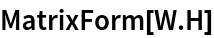Out=Note that elementwise relative errors between the original matrix and reconstructed matrix are small:

 In:=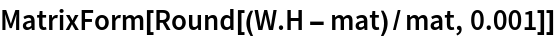Out=### Scope (5)

Here is a random matrix with its first two columns having much larger magnitudes than the rest:

 In:=Out=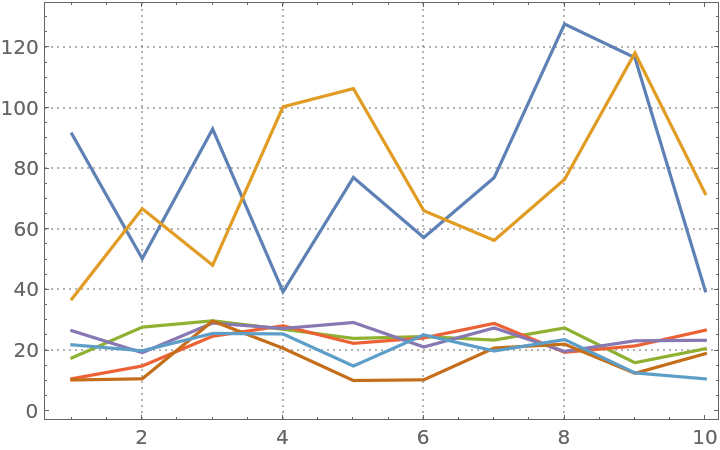Here we compute NNMF factors:

 In:=Find the relative error of the approximation by the matrix factorization:

 In:=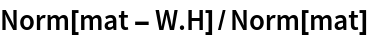Out=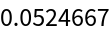Here is the relative error for the first three columns:

 In:=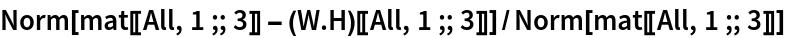Out=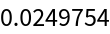Here are comparison plots:

 In:=Out=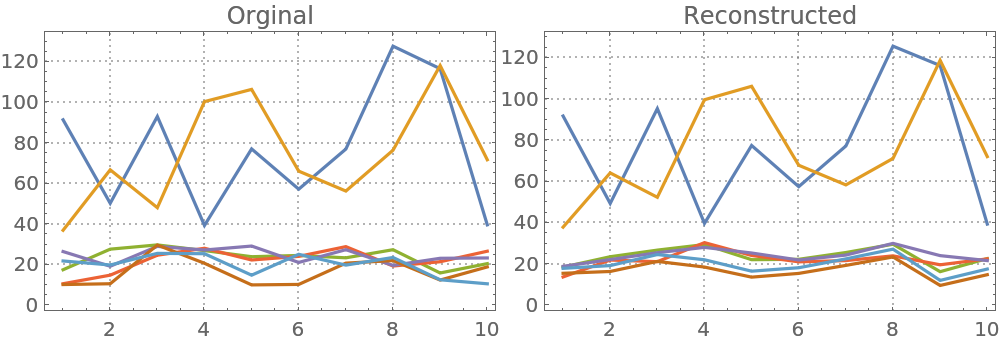### Options (4)

#### Normalization (1)

NNMF factors can be normalized in two ways: (i) the Euclidean norms of the columns of the left factor are all equal to 1; or (ii) the Euclidean norms of the rows of the right factor are all equal to 1. Here is a table that shows NNMF factors for different normalization specifications:

 In:=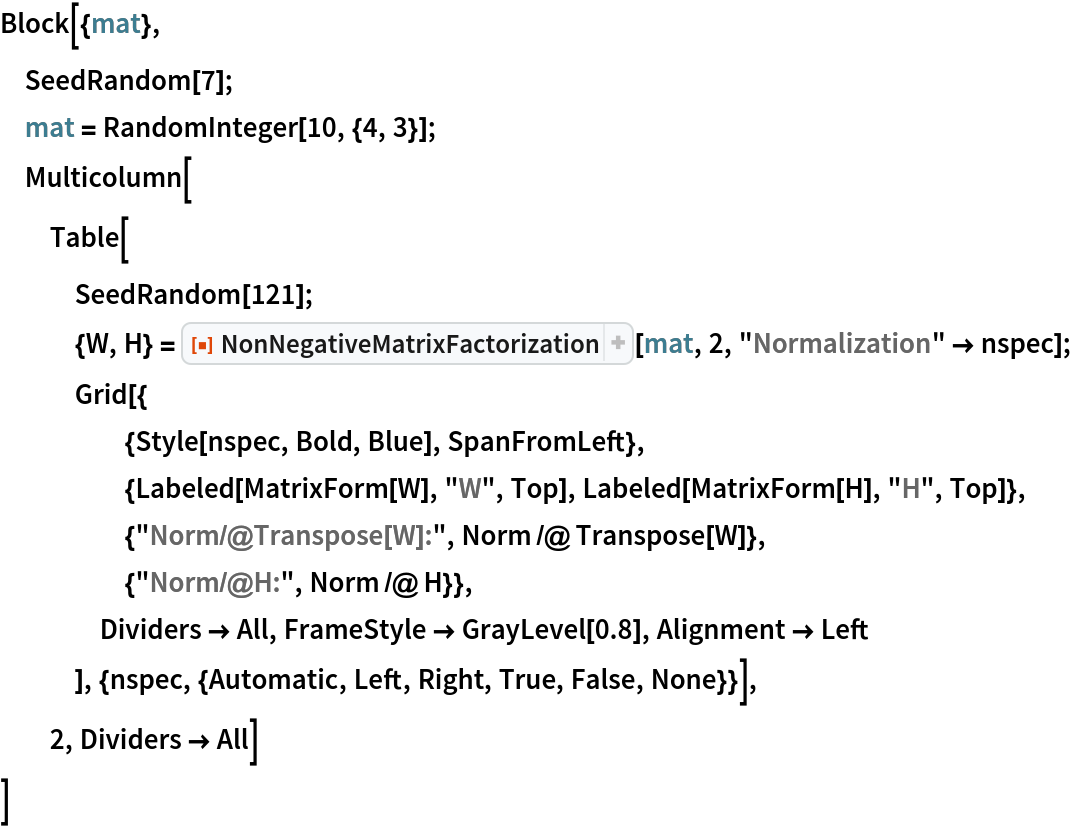Out=#### RegularizationParameter (3)

The implemented NNMF algorithm uses the gradient descent algorithm. The regularization parameter controls the “learning rate” and can have dramatic influence on the number of iteration steps and approximation precision. Compute NNMF with different regularization multiplier parameters:

 In:=Plot the results:

 In:=Out=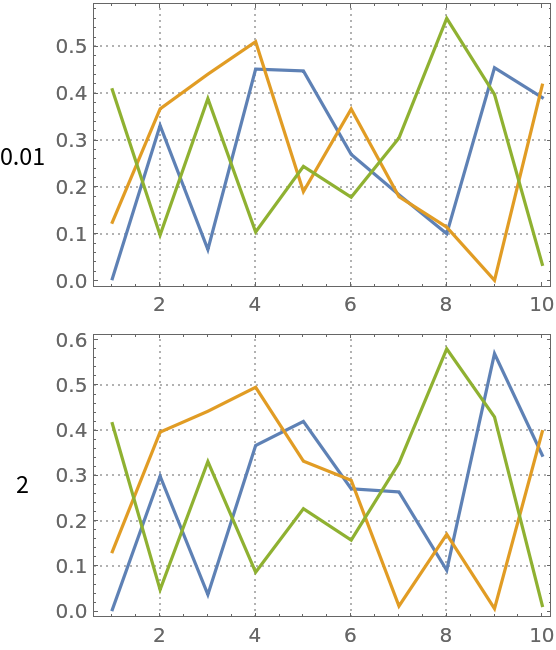Here are the corresponding norms:

 In:=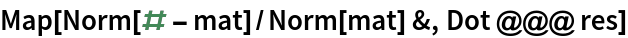Out=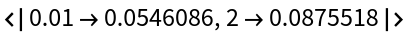### Applications (20)

One of the main motivations for developing NNMF algorithms is the easier interpretation of extracted topics in the framework of latent semantic analysis. The following code illustrates the extraction of topics over a dataset of movie reviews.

 In:=Out=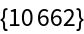Change the labels “positive” and “negative” to have the prefix “tag:”:

 In:=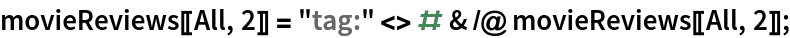Concatenate to each review the corresponding label and create an Association:

 In:=Out=Select movie reviews that are nontrivial:

 In:=Out=Split each review into words and delete stopwords:

 In:=Convert the movie reviews association into a list of ID-word pairs (“long form”):

 In:=Out=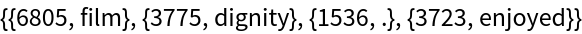Replace each word with its Porter stem:

 In:=Out=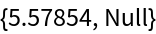Find the frequency of appearance of all unique word stems and pick words that appear in more than 30 reviews:

 In:=Out=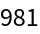Filter the ID-word pairs list to contain only words that are sufficiently popular:

 In:=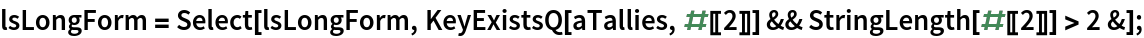Compute an ID-word contingency matrix:

 In:=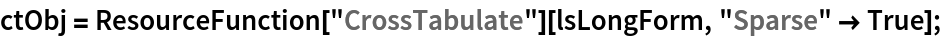Here is a sample of the contingency matrix as a dataset:

 In:=Out=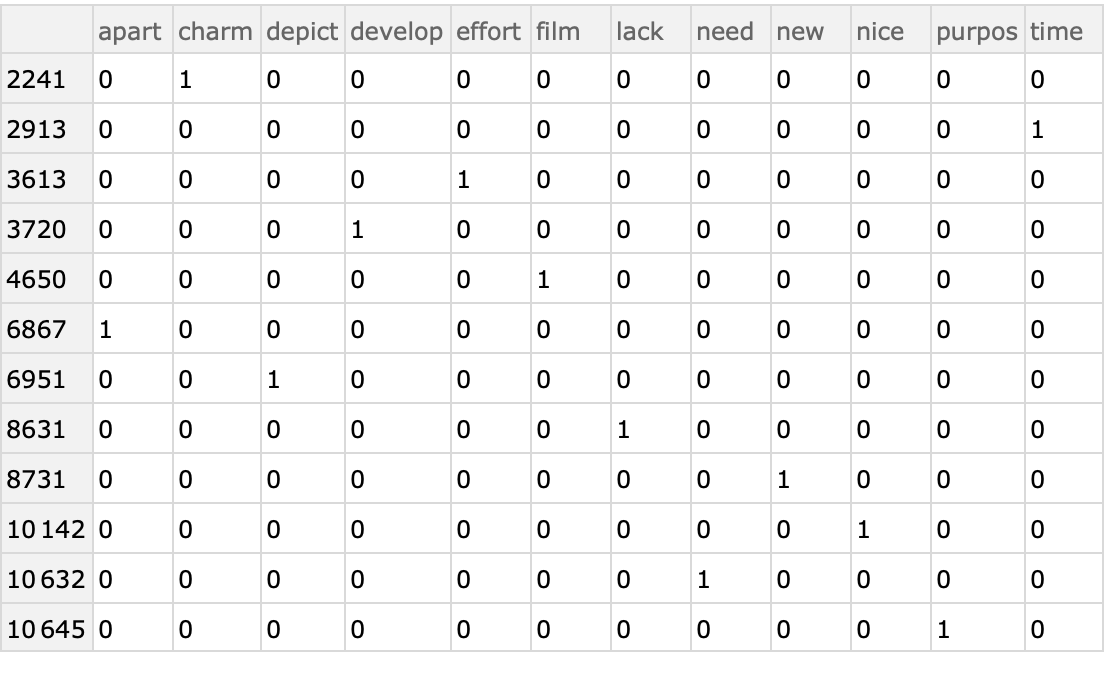Visualize the contingency matrix:

 In:=Out=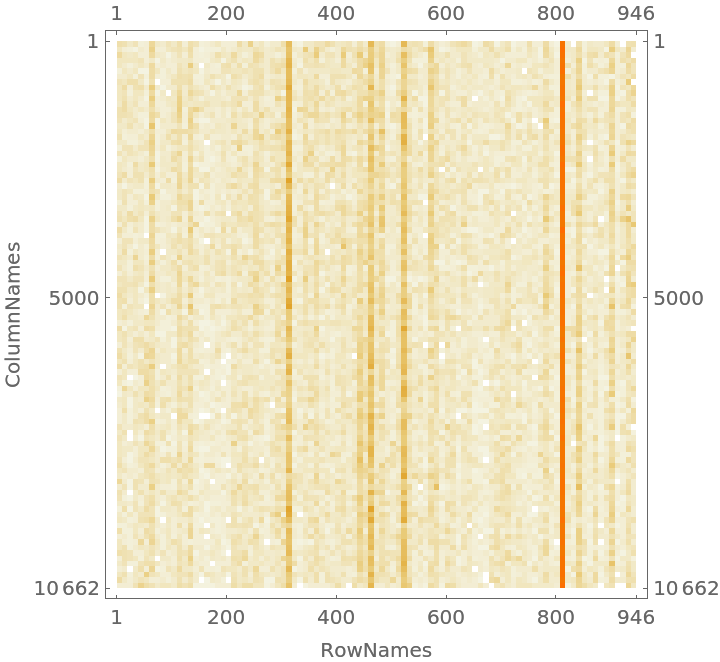Take the contingency sparse matrix:

 In:=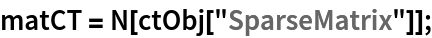Here is a plot generated by the resource function ParetoPrinciplePlot that shows the Pareto principle adherence of the selected popular words:

 In:=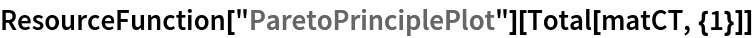Out=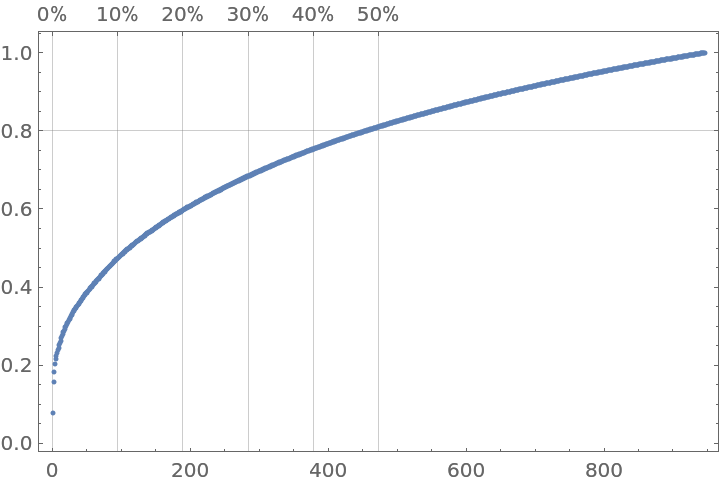Judging from the Pareto plot we should apply the inverse document frequency (IDF) formula:

 In:=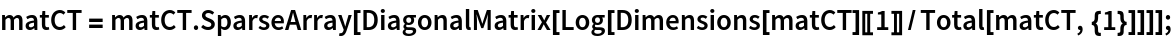Normalize each row with the Euclidean norm:

 In:=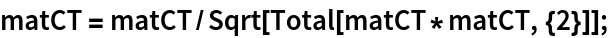In order to get computations faster, we take a sample submatrix:

 In:=Out=Apply NNMF in order to extract 24 topics using 12 maximum iteration steps, normalizing the right factor:

 In:=Out=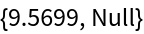Show the extracted topics using the right factor (H):

 In:=Out=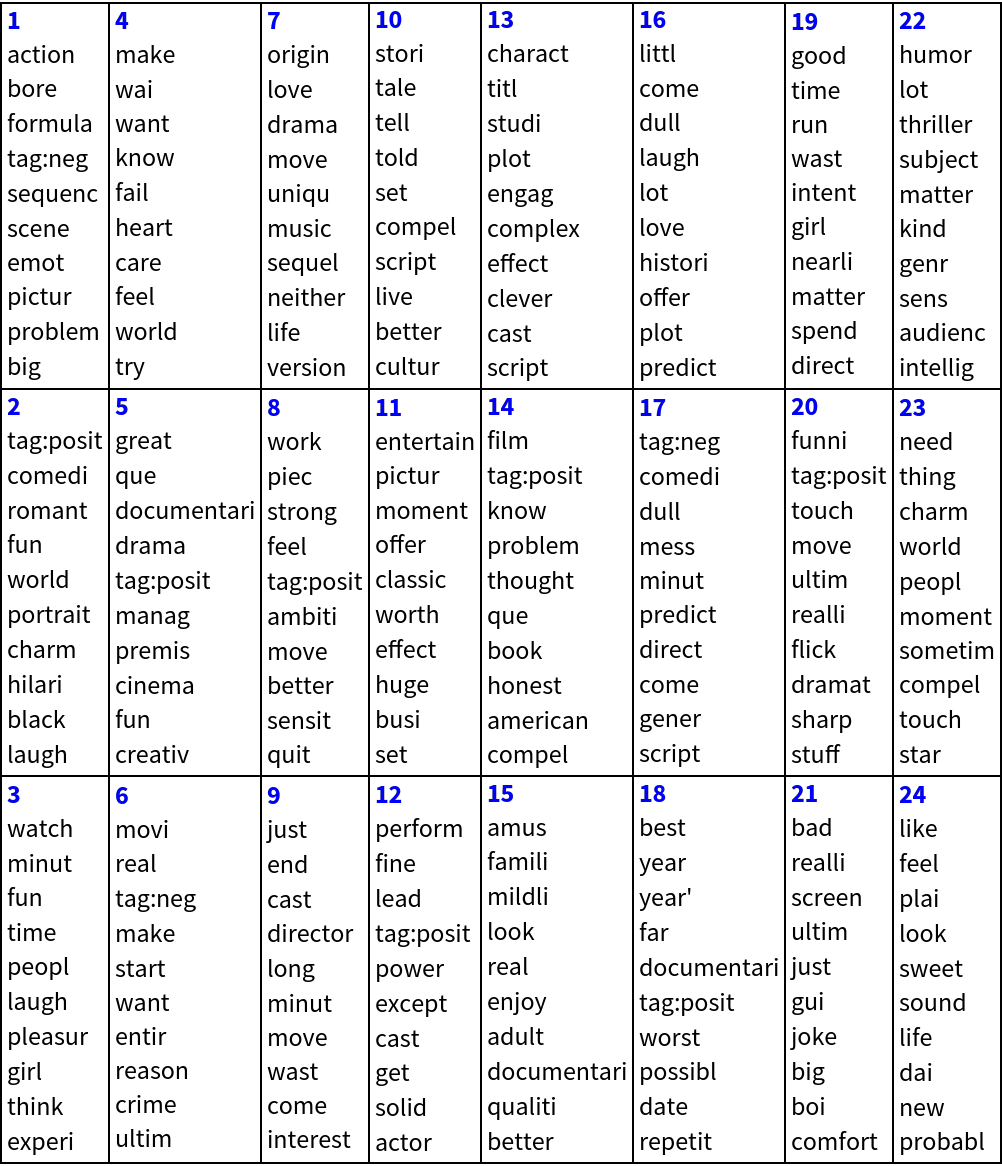Here we show statistical thesaurus entries for random words, selected words and the labels (“tag:positive”,”tag:negative”):

 In:=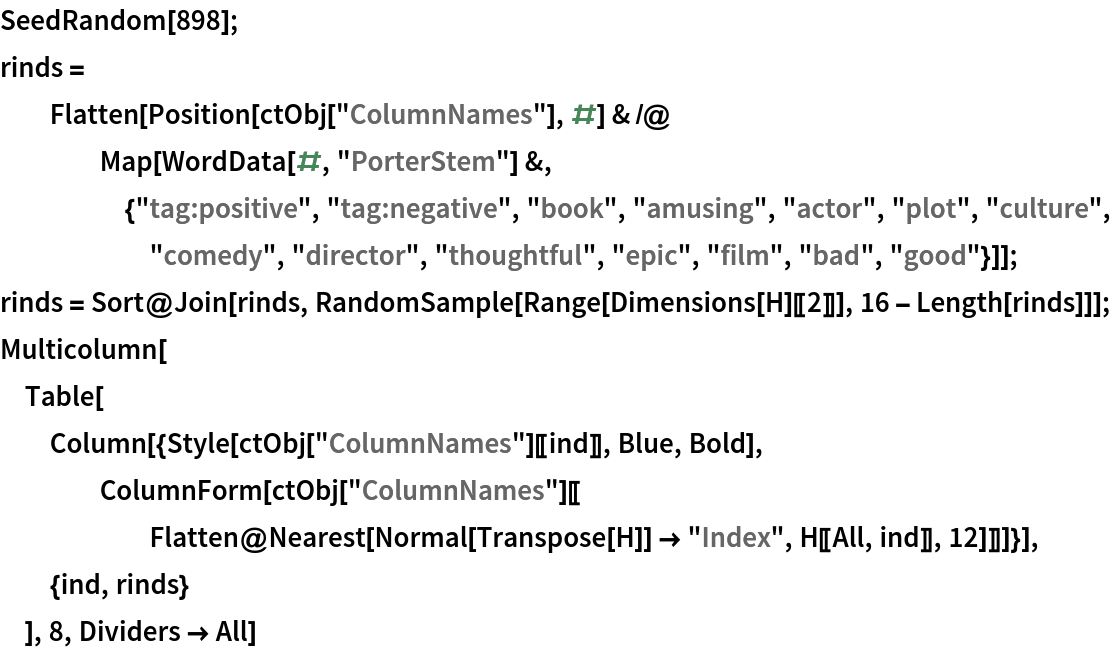Out=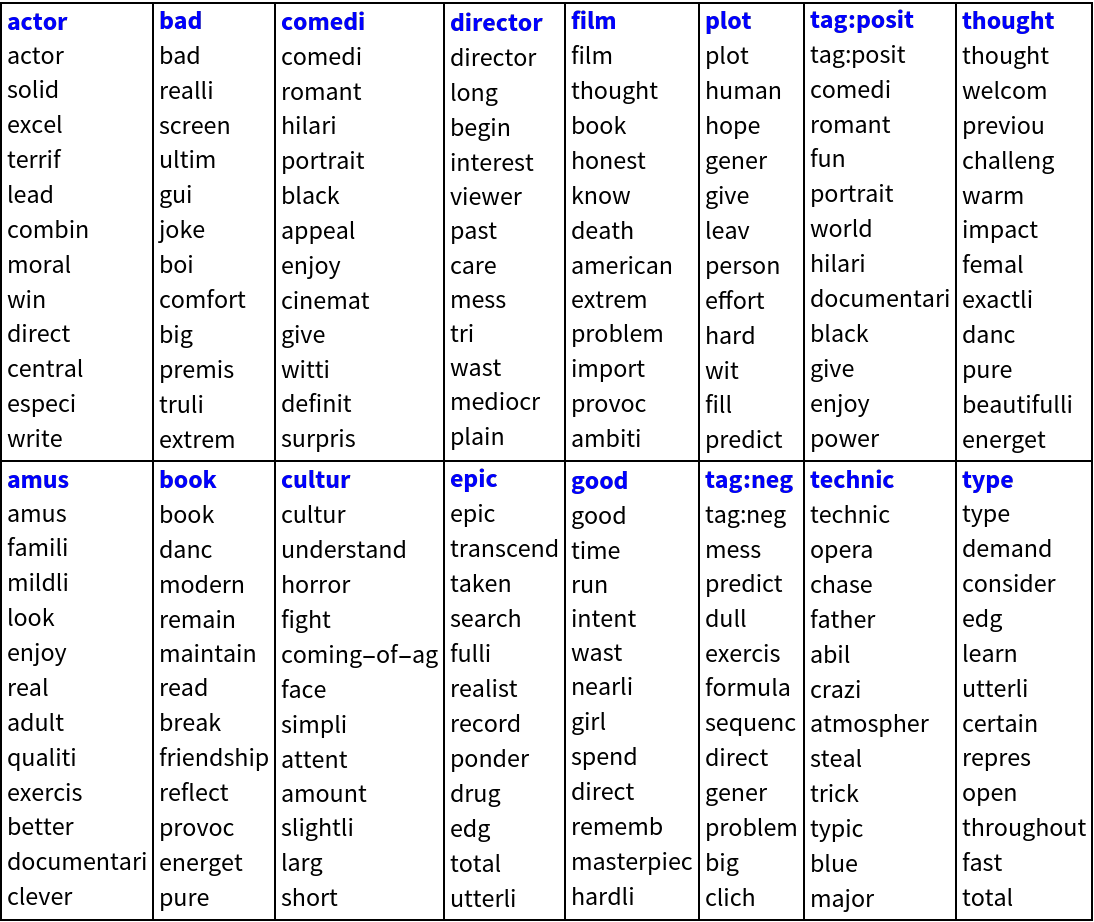### Properties and Relations (2)

NNMF should be compared with the singular value decomposition (SVD) and independent component analysis (ICA).

Generate 3D points:

 In:=Compute matrix factorizations using NNMF, SVD and ICA and make a comparison plots grid (rotate the graphics boxes for better perception):

 In:=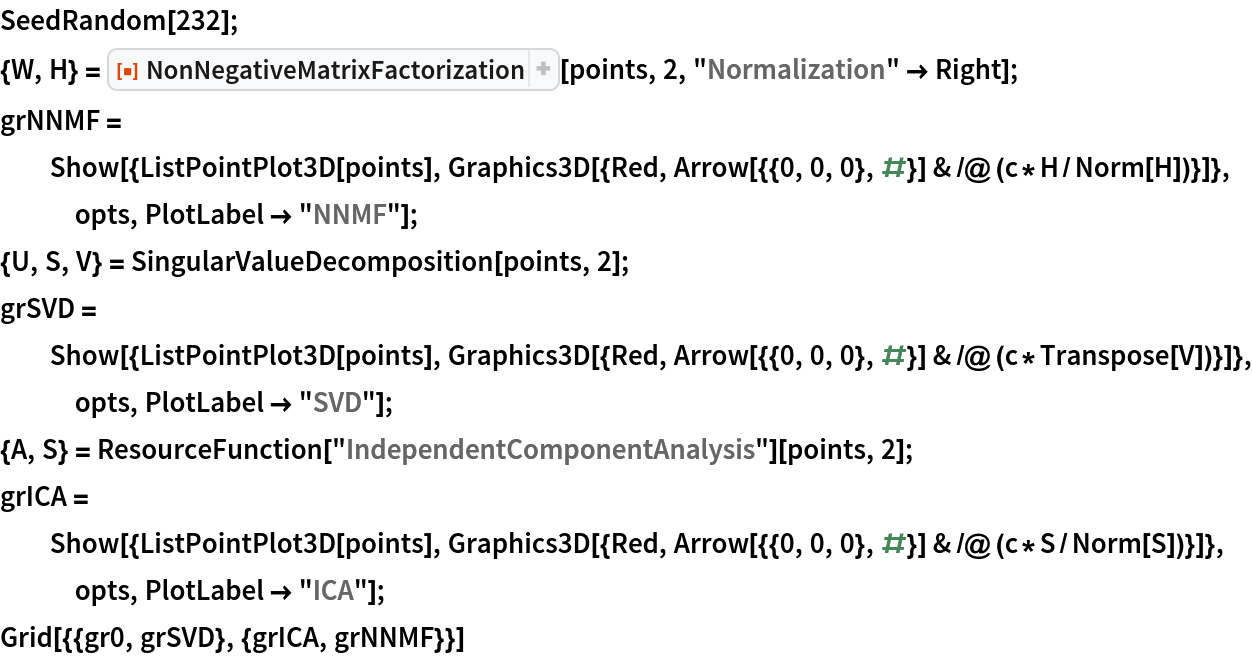Out=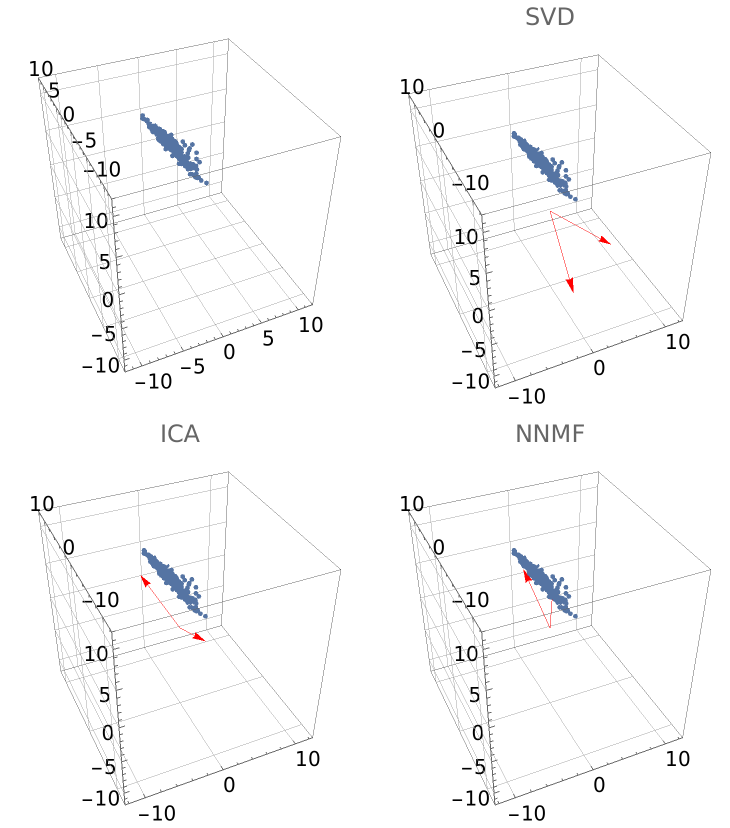Anton Antonov

## Version History

• 1.0.0 – 19 December 2019